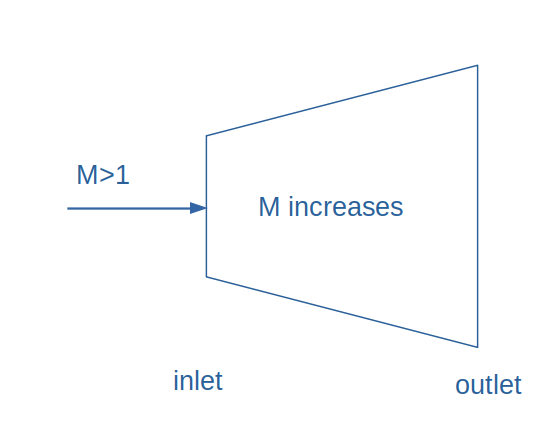Search
•rameez badhurshah

# Rocket nozzles - Mech and Mach

In this blog, we discuss the idea of building up the nozzle for rockets. Wait, what are nozzles? Are there different types of nozzles? Wait, isn't it just the fuel that propels the rocket to supersonic speeds?Finished binge-watching the web series Rocket boys? Still have questions about the mechanics of rocket dynamics. This post is to answer your curb. But, for all the ones who loved "rocket boys", there is another intense movie on a similar topic, Jake Gyllenhaal's starter October Sky. And I highly recommend you to watch this movie for the inspirational story behind a young lad's madness over rockets. Coming back to our blog, the very first baby step in learning about the nozzles is to understand the compressible flow. From there on, we focus on understanding the concept of stagnation state and then move on to the area-velocity relationship to learn about the nozzles. Our final aim is to look into the convergent-divergent nozzles which are the nozzles used in the rockets.

## Compressible Flow

For all major fluid flows especially liquids, we assume the density of the fluid to remain constant during the course of its flow. We call such flows incompressible flows. On the other hand, the flows in which the density change is significant is called compressible flow. High-speed gasses are usually categorized as compressible flow. Compressible flow stems out from the intersection of fluid dynamics and thermodynamics.## Stagnation Properties

When we state a stagnation property, it implies the fluid at that point has zero velocity. Thus, in general, stagnation properties of the gas or fluid are achieved when the fluid is brought to rest adiabatically. The corresponding properties at this state are usually denoted by subscript '0', like stagnation temperature (T0), stagnation pressure (P0), stagnation enthalpy (h0) and so on.

As the velocity becomes zero, the kinetic energy of the fluid gets converted into enthalpy during the stagnation stage, thereby increasing the temperature and pressure of the fluid.

When the stagnation stage is reversible along with being adiabatic, we term it as an isentropic stagnation state.

## Mach number

A significant parameter involved while analysing the compressible flow is the Mach number (M). Mathematically, we define Mach number as the ratio of actual velocity of the fluid to the speed of sound in that fluid. Mach number is highly dependent on the velocity of sound which in turn depends on the atmospheric properties at that point. Thus, an aircraft flying with a constant velocity can have different Mach number depending on the altitude, temperature, pressure and other atmospheric properties. Depending on the Mach number, we have different flow regimes.

The following table illustrates the different flow regimes based on the Mach number## Area velocity relationship

By using the mass balance, energy balance and isentropic flow assumption, we derive one of the most important relationships for determining the geometry of the nozzles/diffusers based on the flow regime. We call this relationship as Area-Velocity relationship and the final form of it is given as:In the above relationship, A, V denote the area and the velocity while dA and dV are their differentials respectively. M is the Mach number. Let us understand the above relation in a better way. For an accelerating flow, dV will be positive while for decelerating flow, dV will be negative. Deceleration of flow is required in the diffusers and since our topic of discussion is nozzles, we will be restricting to accelerating flows only. The below table summarizes the above equation## Nozzle designs

Thus, we now know the equation which governs the nozzle design for the two different types of flows

### 1. Subsonic Nozzle

When the fluid flow is subsonic, to accelerate the flow, it is essential to have a converging area nozzle. This means the cross-sectional area at the exit should be less than that of that at the entry. A simple illustration is shown belowThe maximum velocity that can be achieved at the outlet of a subsonic nozzle is the sonic velocity. It can never exceed the sonic velocity. Thus, this limits the exit velocity at the nozzle.

### 2. Supersonic Nozzle

Supersonic nozzles are designed for supersonic flow wherein the inlet velocity is greater than the sonic velocity and at the exit, much higher Mach speeds can be achieved. The cross-section of such a nozzle is a diverging cross-section.## Convergent-Divergent nozzle

The above two cases are strictly monotonous in nature, which implies with a subsonic inlet velocity, it is impossible to achieve a supersonic velocity at the exit. To solve this puzzle, we use convergent-divergent nozzles (C-D nozzles, de Laval nozzles). In such nozzles under careful operating conditions, it is possible to achieve supersonic outlet velocity even when the inlet is subsonic. The parameter critical to this is the backpressure is crucial in determining if the exit velocity can be achieved as supersonic.## History

Mach number is named in honour of Ernst Waldfried Josef Wenzel Mach for his contributions to the study of shock waves. Naming the actual velocity to the velocity of sound as Mach number was proposed by famous aeronautical engineer Jakob Ackeret. Mach's initial studies were in optics where he performed experiments to study the diffraction, interference and polarization of light in different mediums. Later on, Mach reported the existence of shock waves of conical nature while studying the supersonic motion of a projectile. Mach held the chair for "the history and philosophy of the inductive sciences" between 1895 to 1901, at the University of Vienna.

## Summary

• Compressible flows are the flows in which the density variations are significant

• Mach number relates the actual velocity of the object to the velocity of sound

• Convergent -Divergent nozzles are specially designed to work with subsonic inlets and supersonic outlets.